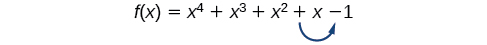# 5.5 Zeros of polynomial functions  (Page 5/14)

 Page 5 / 14

Let $\text{\hspace{0.17em}}f\text{\hspace{0.17em}}$ be a polynomial function with real coefficients, and suppose is a zero of $\text{\hspace{0.17em}}f\left(x\right).\text{\hspace{0.17em}}$ Then, by the Factor Theorem, $\text{\hspace{0.17em}}x-\left(a+bi\right)\text{\hspace{0.17em}}$ is a factor of $\text{\hspace{0.17em}}f\left(x\right).\text{\hspace{0.17em}}$ For $\text{\hspace{0.17em}}f\text{\hspace{0.17em}}$ to have real coefficients, $\text{\hspace{0.17em}}x-\left(a-bi\right)\text{\hspace{0.17em}}$ must also be a factor of $\text{\hspace{0.17em}}f\left(x\right).\text{\hspace{0.17em}}$ This is true because any factor other than $\text{\hspace{0.17em}}x-\left(a-bi\right),\text{\hspace{0.17em}}$ when multiplied by $\text{\hspace{0.17em}}x-\left(a+bi\right),\text{\hspace{0.17em}}$ will leave imaginary components in the product. Only multiplication with conjugate pairs will eliminate the imaginary parts and result in real coefficients. In other words, if a polynomial function $\text{\hspace{0.17em}}f\text{\hspace{0.17em}}$ with real coefficients has a complex zero $\text{\hspace{0.17em}}a+bi,\text{\hspace{0.17em}}$ then the complex conjugate $\text{\hspace{0.17em}}a-bi\text{\hspace{0.17em}}$ must also be a zero of $\text{\hspace{0.17em}}f\left(x\right).\text{\hspace{0.17em}}$ This is called the Complex Conjugate Theorem .

## Complex conjugate theorem

According to the Linear Factorization Theorem     , a polynomial function will have the same number of factors as its degree, and each factor will be in the form $\text{\hspace{0.17em}}\left(x-c\right)$ , where $\text{\hspace{0.17em}}c\text{\hspace{0.17em}}$ is a complex number.

If the polynomial function $\text{\hspace{0.17em}}f\text{\hspace{0.17em}}$ has real coefficients and a complex zero in the form $\text{\hspace{0.17em}}a+bi,\text{\hspace{0.17em}}$ then the complex conjugate of the zero, $\text{\hspace{0.17em}}a-bi,\text{\hspace{0.17em}}$ is also a zero.

Given the zeros of a polynomial function $\text{\hspace{0.17em}}f\text{\hspace{0.17em}}$ and a point ( c , f ( c )) on the graph of $\text{\hspace{0.17em}}f,\text{\hspace{0.17em}}$ use the Linear Factorization Theorem to find the polynomial function.

1. Use the zeros to construct the linear factors of the polynomial.
2. Multiply the linear factors to expand the polynomial.
3. Substitute $\text{\hspace{0.17em}}\left(c,f\left(c\right)\right)\text{\hspace{0.17em}}$ into the function to determine the leading coefficient.
4. Simplify.

## Using the linear factorization theorem to find a polynomial with given zeros

Find a fourth degree polynomial with real coefficients that has zeros of –3, 2, $\text{\hspace{0.17em}}i,\text{\hspace{0.17em}}$ such that $\text{\hspace{0.17em}}f\left(-2\right)=100.\text{\hspace{0.17em}}$

Because $\text{\hspace{0.17em}}x=i\text{\hspace{0.17em}}$ is a zero, by the Complex Conjugate Theorem $\text{\hspace{0.17em}}x=–i\text{\hspace{0.17em}}$ is also a zero. The polynomial must have factors of $\text{\hspace{0.17em}}\left(x+3\right),\text{\hspace{0.17em}}\left(x-2\right),\text{\hspace{0.17em}}\left(x-i\right),\text{\hspace{0.17em}}$ and $\text{\hspace{0.17em}}\left(x+i\right).\text{\hspace{0.17em}}$ Since we are looking for a degree 4 polynomial, and now have four zeros, we have all four factors. Let’s begin by multiplying these factors.

$\begin{array}{ccc}\hfill f\left(x\right)& =& a\left(x+3\right)\left(x-2\right)\left(x-i\right)\left(x+i\right)\hfill \\ \hfill f\left(x\right)& =& a\left({x}^{2}+x-6\right)\left({x}^{2}+1\right)\hfill \\ \hfill f\left(x\right)& =& a\left({x}^{4}+{x}^{3}-5{x}^{2}+x-6\right)\hfill \end{array}$

We need to find a to ensure $\text{\hspace{0.17em}}f\left(–2\right)=100.\text{\hspace{0.17em}}$ Substitute and $\text{\hspace{0.17em}}f\left(2\right)=100\text{\hspace{0.17em}}$ into $\text{\hspace{0.17em}}f\left(x\right).\text{\hspace{0.17em}}$

$\begin{array}{ccc}\hfill 100& =& a\left({\left(-2\right)}^{4}+{\left(-2\right)}^{3}-5{\left(-2\right)}^{2}+\left(-2\right)-6\right)\hfill \\ \hfill 100& =& a\left(-20\right)\hfill \\ \hfill -5& =& a\hfill \end{array}$

So the polynomial function is

$f\left(x\right)=-5\left({x}^{4}+{x}^{3}-5{x}^{2}+x-6\right)$

or

$f\left(x\right)=-5{x}^{4}-5{x}^{3}+25{x}^{2}-5x+30$

If $\text{\hspace{0.17em}}2+3i\text{\hspace{0.17em}}$ were given as a zero of a polynomial with real coefficients, would $\text{\hspace{0.17em}}2-3i\text{\hspace{0.17em}}$ also need to be a zero?

Yes. When any complex number with an imaginary component is given as a zero of a polynomial with real coefficients, the conjugate must also be a zero of the polynomial.

Find a third degree polynomial with real coefficients that has zeros of 5 and $\text{\hspace{0.17em}}-2i\text{\hspace{0.17em}}$ such that $\text{\hspace{0.17em}}f\left(1\right)=10.\text{\hspace{0.17em}}$

$f\left(x\right)=-\frac{1}{2}{x}^{3}+\frac{5}{2}{x}^{2}-2x+10$

## Using descartes’ rule of signs

There is a straightforward way to determine the possible numbers of positive and negative real zeros for any polynomial function. If the polynomial is written in descending order, Descartes’ Rule of Signs tells us of a relationship between the number of sign changes in $\text{\hspace{0.17em}}f\left(x\right)\text{\hspace{0.17em}}$ and the number of positive real zeros. For example, the polynomial function below has one sign change.This tells us that the function must have 1 positive real zero.

root under 3-root under 2 by 5 y square
The sum of the first n terms of a certain series is 2^n-1, Show that , this series is Geometric and Find the formula of the n^th
cosA\1+sinA=secA-tanA
why two x + seven is equal to nineteen.
The numbers cannot be combined with the x
Othman
2x + 7 =19
humberto
2x +7=19. 2x=19 - 7 2x=12 x=6
Yvonne
because x is 6
SAIDI
what is the best practice that will address the issue on this topic? anyone who can help me. i'm working on my action research.
simplify each radical by removing as many factors as possible (a) √75
how is infinity bidder from undefined?
what is the value of x in 4x-2+3
give the complete question
Shanky
4x=3-2 4x=1 x=1+4 x=5 5x
Olaiya
hi can you give another equation I'd like to solve it
Daniel
what is the value of x in 4x-2+3
Olaiya
if 4x-2+3 = 0 then 4x = 2-3 4x = -1 x = -(1÷4) is the answer.
Jacob
4x-2+3 4x=-3+2 4×=-1 4×/4=-1/4
LUTHO
then x=-1/4
LUTHO
4x-2+3 4x=-3+2 4x=-1 4x÷4=-1÷4 x=-1÷4
LUTHO
A research student is working with a culture of bacteria that doubles in size every twenty minutes. The initial population count was  1350  bacteria. Rounding to five significant digits, write an exponential equation representing this situation. To the nearest whole number, what is the population size after  3  hours?
v=lbh calculate the volume if i.l=5cm, b=2cm ,h=3cm
Need help with math
Peya
can you help me on this topic of Geometry if l help you
litshani
( cosec Q _ cot Q ) whole spuare = 1_cosQ / 1+cosQ
A guy wire for a suspension bridge runs from the ground diagonally to the top of the closest pylon to make a triangle. We can use the Pythagorean Theorem to find the length of guy wire needed. The square of the distance between the wire on the ground and the pylon on the ground is 90,000 feet. The square of the height of the pylon is 160,000 feet. So, the length of the guy wire can be found by evaluating √(90000+160000). What is the length of the guy wire?
the indicated sum of a sequence is known as
how do I attempted a trig number as a starter
cos 18 ____ sin 72 evaluateByByBy Michael SagBy OpenStaxBy Edward BitonBy Charles JumperBy Yasser IbrahimBy Karen GowdeyBy Madison ChristianByBy Rohini AjayBy OpenStax